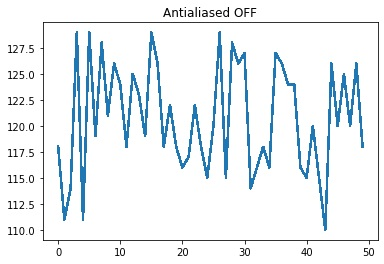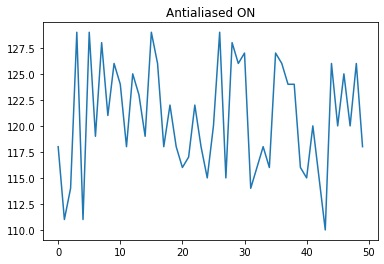# Python | Antialiasing in Plotting

Python | Antialiasing in Plotting: In this article, we are going to learn about the antialiasing in plotting and its Python implementation.
Submitted by Anuj Singh, on July 11, 2020

Antialiasing is another important feature of Matplotlib and in this article, we will review how to use this functionality. pyplot.antialiased() is an inbuilt function in matplotlib.pyplot which performs our required operation.

Syntax:

```line, = plt.plot(x, y, '-')
line.set_antialiased(False) # turn off antialiasing
```## Python code for antialiasing in plotting

```# Data Visualization using Python
# Antialiasing

import numpy as np
import matplotlib.pyplot as plt

x = np.arange(50)
y = 60 + np.random.randint(50,70,50)

plt.figure()
plt.title('Antialiased OFF')
line, = plt.plot(x, y)
# turn off antialiasing
line.set_antialiased(False)

plt.figure()
plt.title('Antialiased ON')
line, = plt.plot(x, y)
# turn on antialiasing
line.set_antialiased(True)
```

Output:

```Output is as figure
```